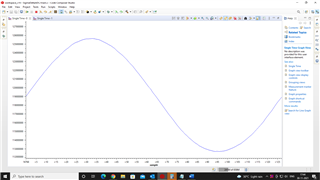#### The TI E2E™ design support forums will undergo maintenance from Sept. 28 to Oct. 2. If you need design support during this time, contact your TI representative or open a new support request with our customer support center.

This thread has been locked.

If you have a related question, please click the "Ask a related question" button in the top right corner. The newly created question will be automatically linked to this question.

# MSP430F6736A: Finding voltage value from SigmaDelta ADC output

Part Number: MSP430F6736A

Hi,
I am using Sigma Delta ADC in MSP430F6736A to convert an Input signal of 13.5Hz with 100mVpp amplitude and 50mV offset.

SMCLK is used as SD24_B clock source and OSR =607

But output has more than 24 bits . And when I am converting it to voltage using

voltage[i]=(float)(results[i]*3/con);             //con=0x1000000(2^24)

Voltage[i] is around 20 but the input signal is 100mVpp with 50mV offset. When Voltage is calculated after removing offset from results[i]  result voltage is between -200.36mV and 200.756mV

Please help me with suggestions on what could be going on here.

Thanks & regards,

Anusree

• Hi

The SD24 is a different input ADC it will minus DC voltage internally.

The Full-sale output depends on your OSR.  Con is not 24bit value base on your over sampling rate.

• Thanks for the reply.

i am getting a 28 bit output.I recalculated the output using 2^28.

But the value obtained is 1.309 and 1.415 for a sqare signal of 13.5Hz with 100mVpp amplitude and 50mV offset.

• What is the value of the ADC in the register? Whether it can correctly reflect the voltage collected by ADC. Because I don't know what calculation you added in the software. What is the type of the variable of results[i] and voltage[i]?

• results[] is of type unsigned long and voltage : float

I think the register is able to reflect the voltage collected by the ADC.
When I plot the values I get the sine wave and the calculated frequency is almost 13.5 Hz and Peak to peak value is almost 100mV(1.415 -1.309)unsigned int resultsH[Num_of_Results], resultsL[Num_of_Results];
long unsigned int results[Num_of_Results],FR[Num_of_Results];

case SD24BIV_SD24IFG0:
resultsH[index] = SD24BMEMH0;// SD24MEM0 IFG
resultsL[index] = SD24BMEML0;// SD24MEM0 IFG
if (++index == Num_of_Results)
{
for(i=0;i<Num_of_Results;i++)
{
FR[i]=resultsH[i];
results[i]=(FR[i]<<16) |(resultsL[i]) ;
mean+=results[i]; // To find Mean(Zero crossing)

voltage[i]=(float)(results[i]*3)/con; // voltage value of SigmaDelta ADC Output
}

mean=mean/Num_of_Results; //To find Mean(Zero crossing)
for(i=0;i<Num_of_Results;i++)
{
r[i]= results[i]-mean; //Removing the offset
voltage_r[i]=(float)(r[i]*3)/con; // voltage value of shifted signal
}
}

When I remove the mean value from the results[] and calculate the voltage it is almost in the range -50mV to 50mV.

• Hi Anusree,

Got your questions, I can join and try to help you quickly.

Thanks!

Best Regards

Johnson

• Have you watch the mean value in debug mode? You could add all the variables in the watch expression to find which intermediate variables is not performed as excepted.

**Attention** This is a public forum